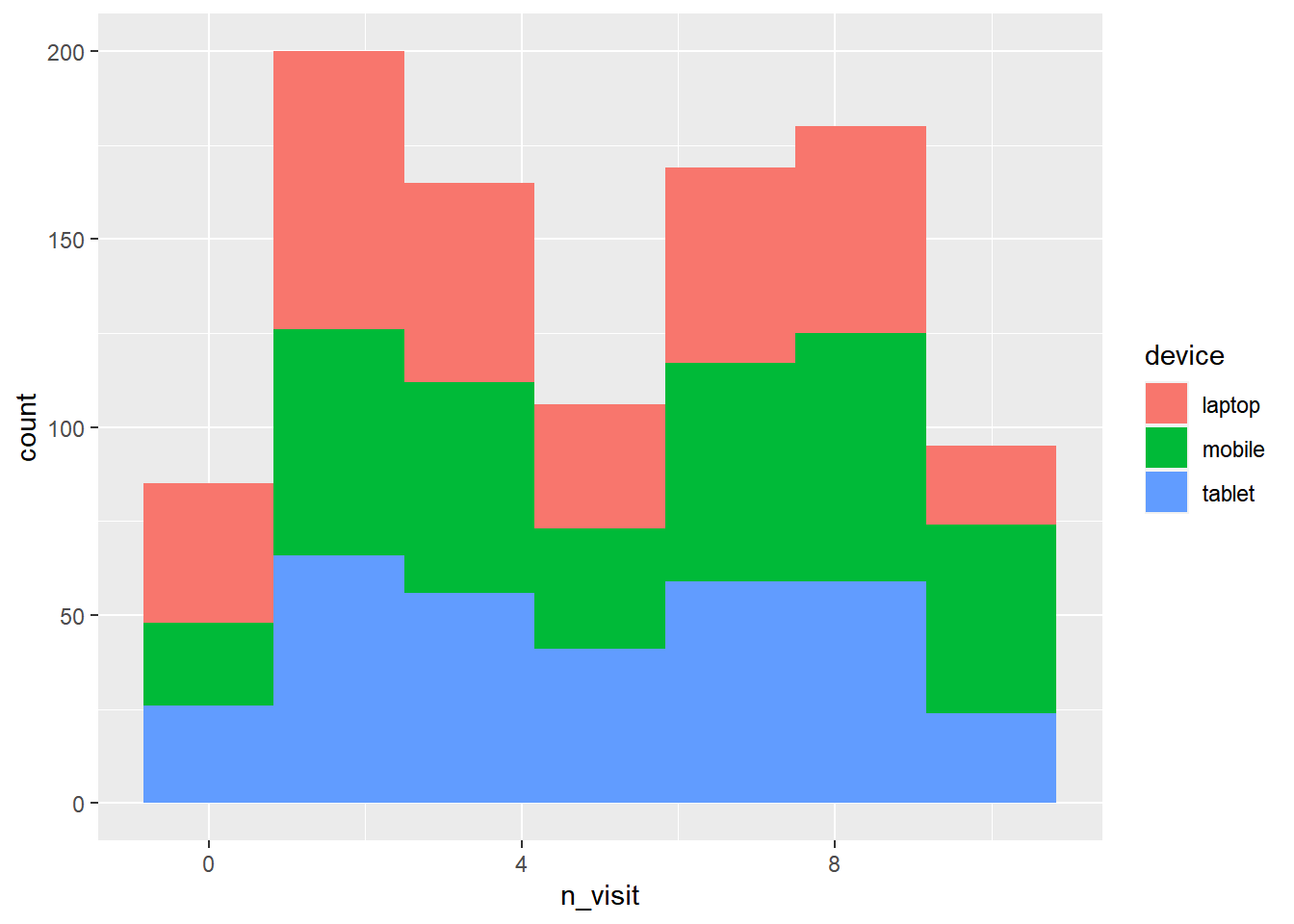# Chapter 10 Histograms

## 10.1 Introduction

In this chapter, we will learn to

• build histogram
• specify bins
• modify
• color
• fill
• alpha
• bin width
• line type
• line size
• map aesthetics to variables

A histogram is a plot that can be used to examine the shape and spread of continuous data. It looks very similar to a bar graph and can be used to detect outliers and skewness in data. The histogram graphically shows the following:

• center (location) of the data
• spread (dispersion) of the data
• skewness
• outliers
• presence of multiple modes

To construct a histogram, the data is split into intervals called bins. The intervals may or may not be equal sized. For each bin, the number of data points that fall into it are counted (frequency). The Y axis of the histogram represents the frequency and the X axis represents the variable.

## 10.2 Data

``````ecom <- readr::read_csv('https://raw.githubusercontent.com/rsquaredacademy/datasets/master/web.csv')
ecom``````
``````## # A tibble: 1,000 x 11
##       id referrer device bouncers n_visit n_pages duration country      purchase
##    <dbl> <chr>    <chr>  <lgl>      <dbl>   <dbl>    <dbl> <chr>        <lgl>
##  1     1 google   laptop TRUE          10       1      693 Czech Repub~ FALSE
##  2     2 yahoo    tablet TRUE           9       1      459 Yemen        FALSE
##  3     3 direct   laptop TRUE           0       1      996 Brazil       FALSE
##  4     4 bing     tablet FALSE          3      18      468 China        TRUE
##  5     5 yahoo    mobile TRUE           9       1      955 Poland       FALSE
##  6     6 yahoo    laptop FALSE          5       5      135 South Africa FALSE
##  7     7 yahoo    mobile TRUE          10       1       75 Bangladesh   FALSE
##  8     8 direct   mobile TRUE          10       1      908 Indonesia    FALSE
##  9     9 bing     mobile FALSE          3      19      209 Netherlands  FALSE
## 10    10 google   mobile TRUE           6       1      208 Czech Repub~ FALSE
## # ... with 990 more rows, and 2 more variables: order_items <dbl>,
## #   order_value <dbl>``````

### 10.2.1 Data Dictionary

• id: row id
• referrer: referrer website/search engine
• os: operating system
• browser: browser
• device: device used to visit the website
• n_pages: number of pages visited
• duration: time spent on the website (in seconds)
• repeat: frequency of visits
• country: country of origin
• purchase: whether visitor purchased
• order_value: order value of visitor (in dollars)

## 10.3 Plot

To create a histogram, we will use `geom_histogram()` and specify the variable name within `aes()`. In the below example, we create histogram of the variable `n_visit`.

``````ggplot(ecom) +
geom_histogram(aes(n_visit))``````
``## `stat_bin()` using `bins = 30`. Pick better value with `binwidth`.``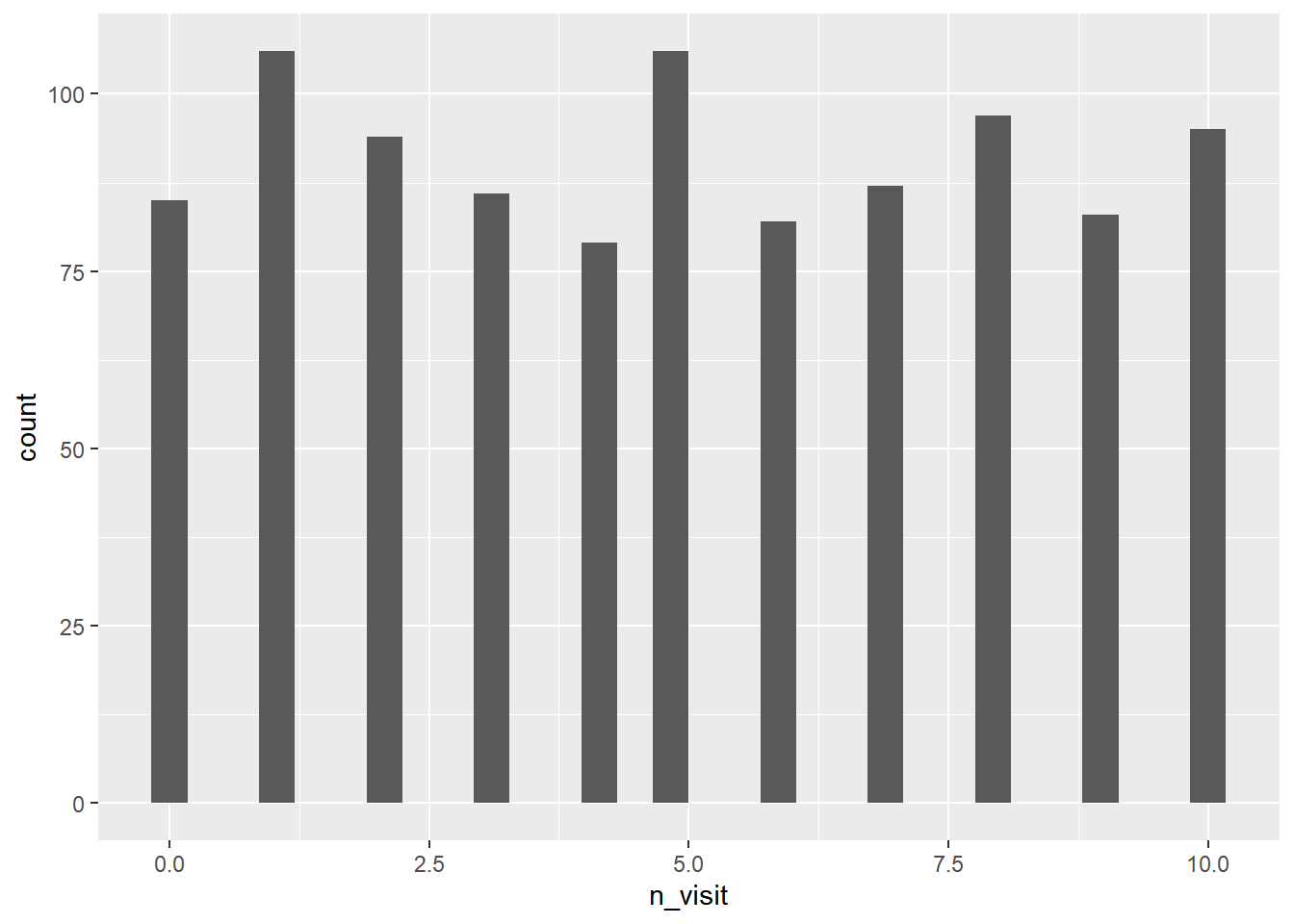### 10.3.1 Specify Bins

The default number of bins in ggplot2 is `30`. You can modify the number of bins using the `bins` argument. In the below example, we create a histogram with 7 bins.

``````ggplot(ecom) +
geom_histogram(aes(n_visit), bins = 7)``````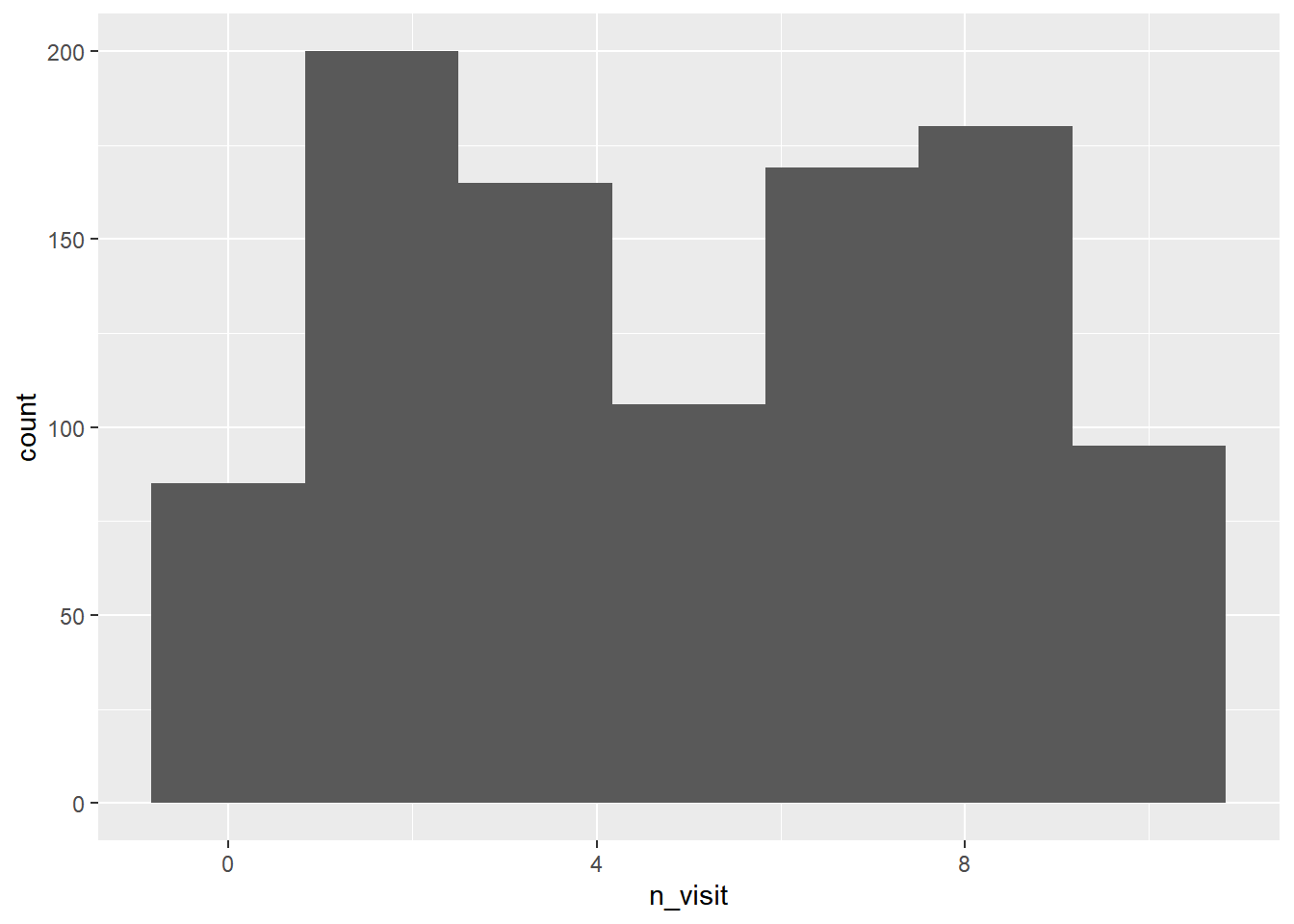## 10.4 Aesthetics

Now that we know how to create a histogram, let us learn to modify its appearance. We will begin with the background color. Use the `fill` argument to modify the background color of the histogram. In the below case, we change the color of the histogram to ‘blue’.

``````ggplot(ecom) +
geom_histogram(aes(n_visit), bins = 7, fill = 'blue')``````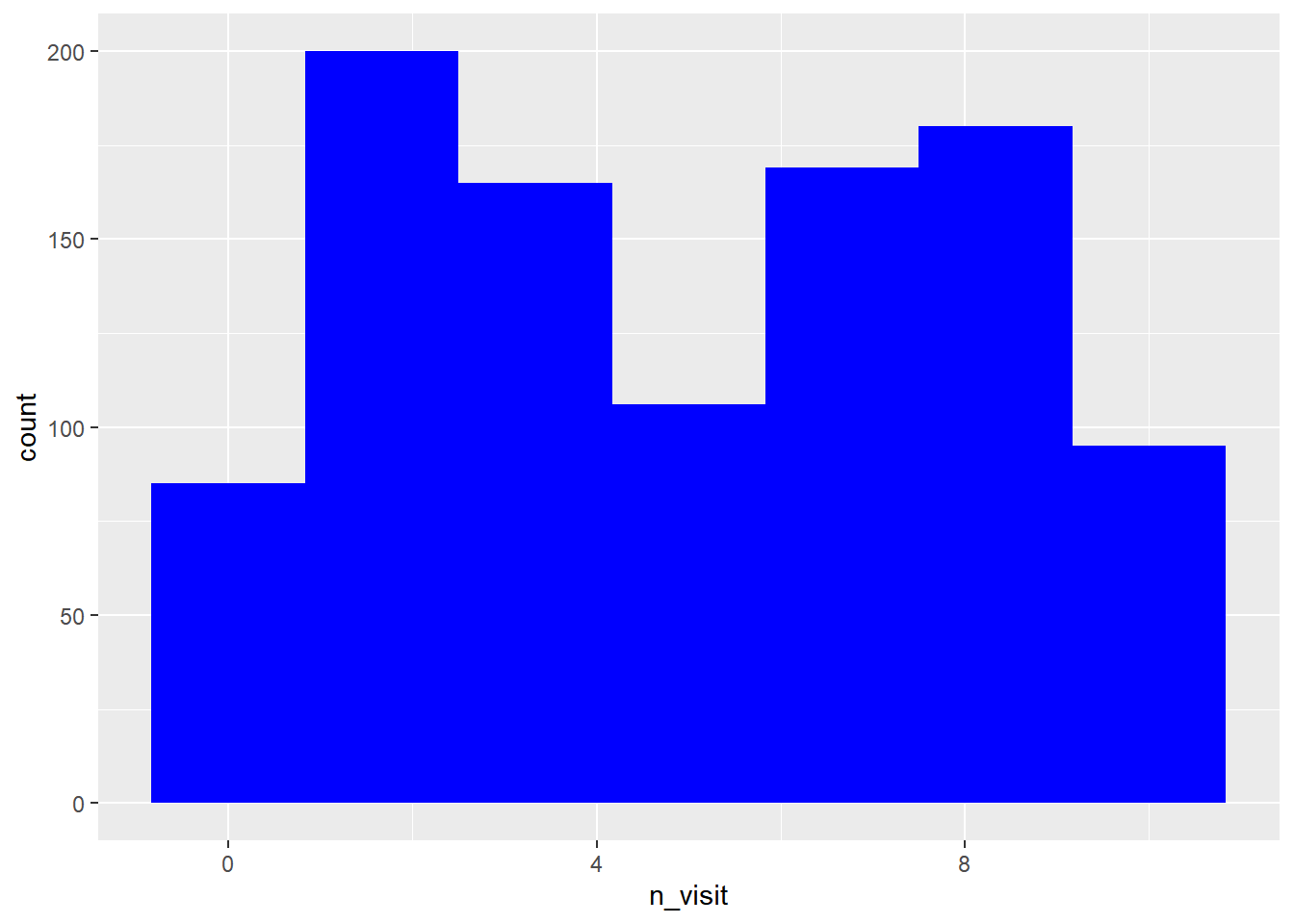As we have learnt before, the transparency of the background color can be modified using the `alpha` argument. It can take any value between `0` and `1`.

``````ggplot(ecom) +
geom_histogram(aes(n_visit), bins = 7, fill = 'blue', alpha = 0.3)``````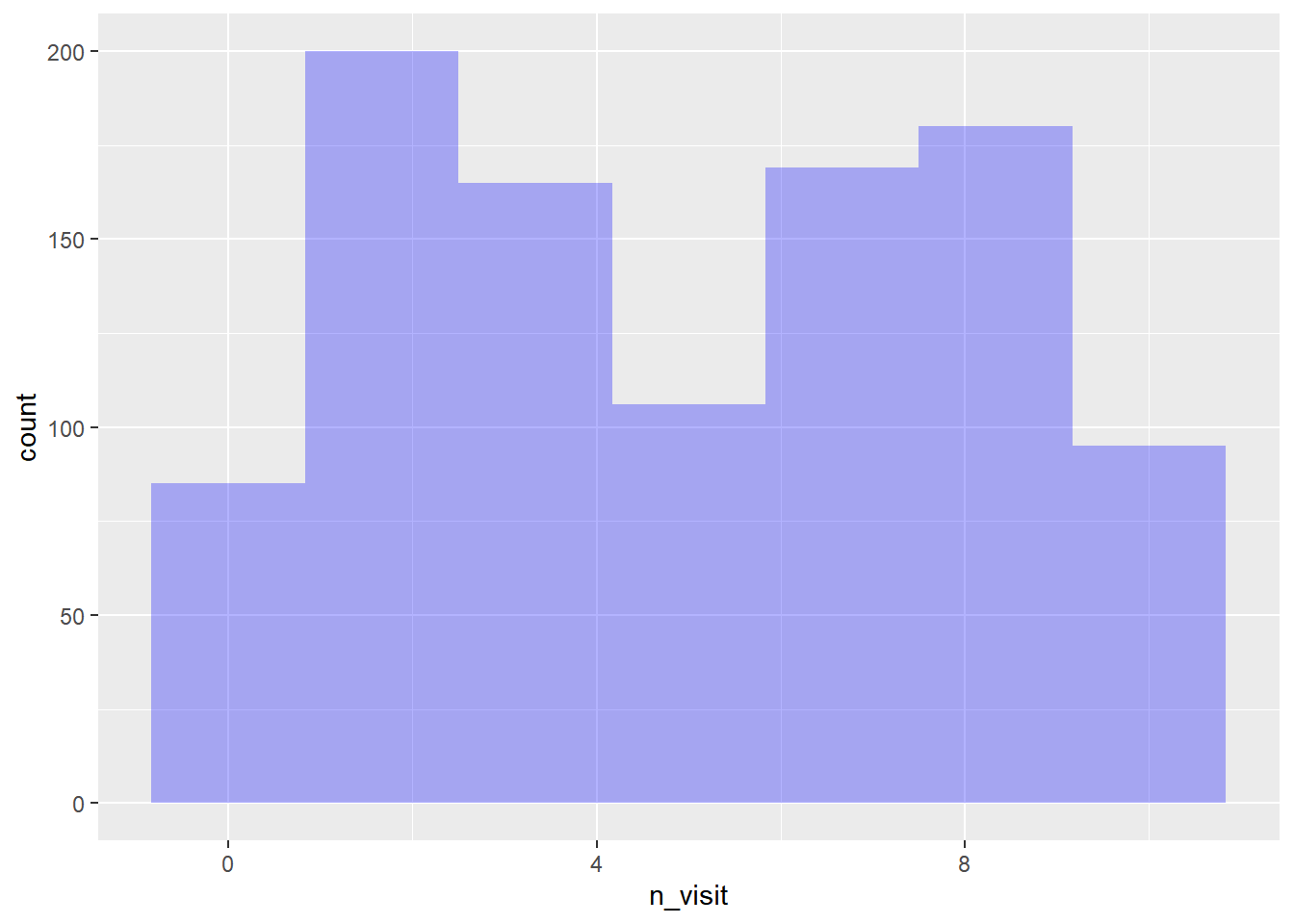The color of the histogram border can be modified using the `color` argument. The color can be specified either using its name or the associated hex code.

``````ggplot(ecom) +
geom_histogram(aes(n_visit), bins = 7, fill = 'white', color = 'blue')``````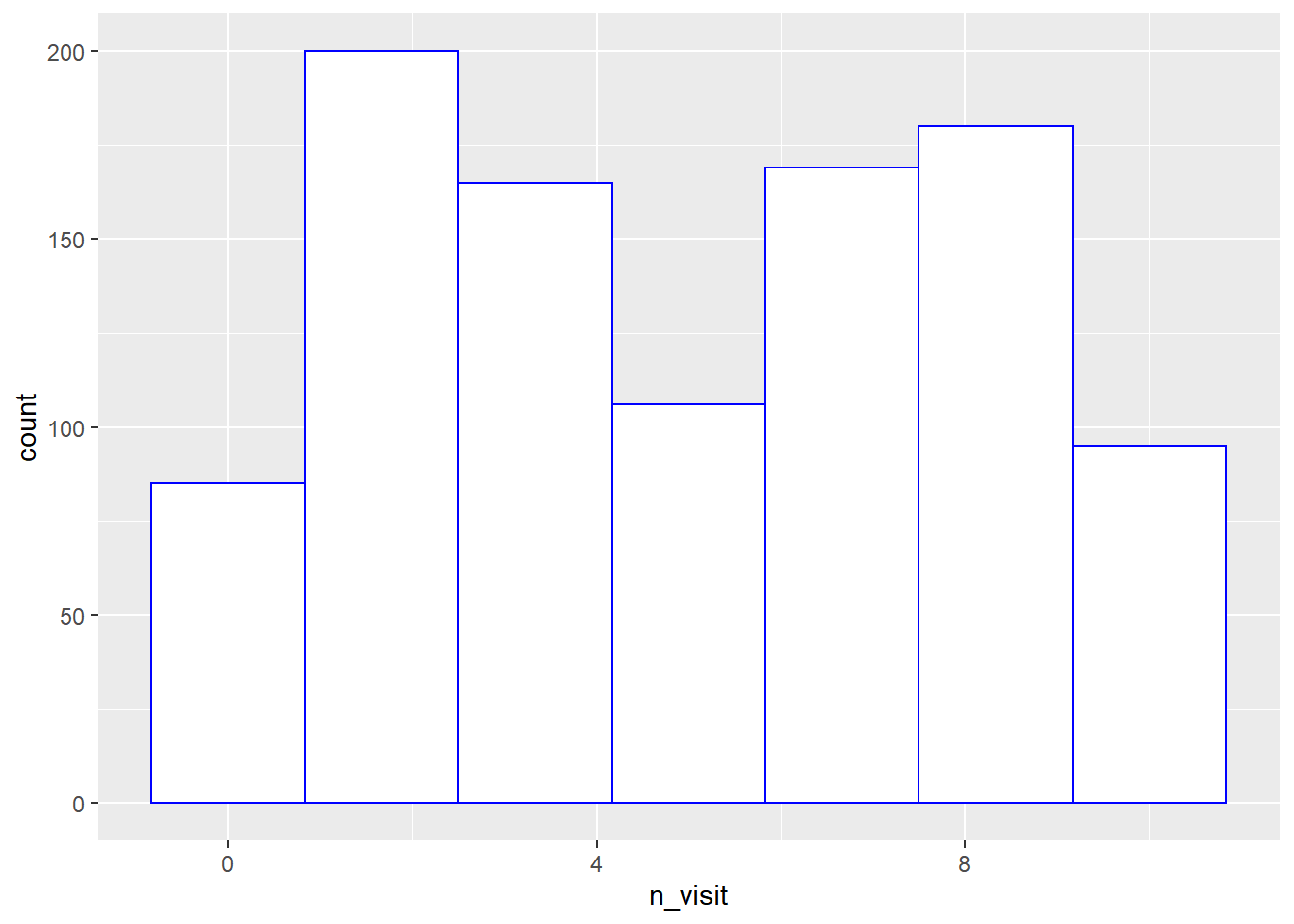## 10.5 Putting it all together…

Let us modify the bins, the background and border color of the histogram in the below example.

``````ggplot(ecom) +
geom_histogram(aes(n_visit), bins = 7, fill = 'blue', color = 'white')``````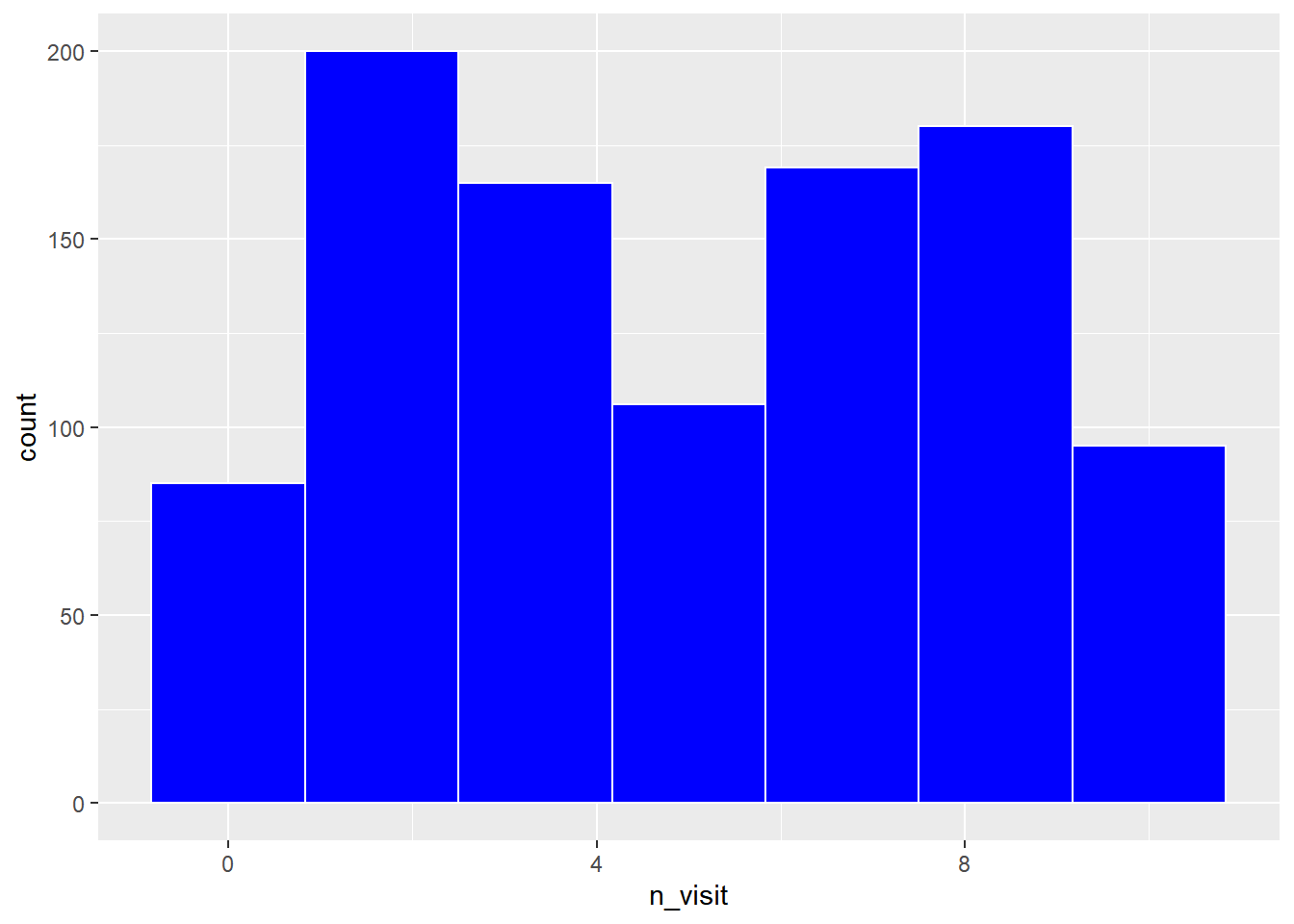## 10.6 Bin Width

Another way to control the number of bins in a histogram is by using the `binwidth` argument. In this case, we specify the width of the bins instead of the number of bins. As you can see, in the below example, we do not use the `bins` argument when using the `binwidth` argument. You can use either of them but not both.

``````ggplot(ecom) +
geom_histogram(aes(n_visit), binwidth = 2, fill = 'blue', color = 'black')``````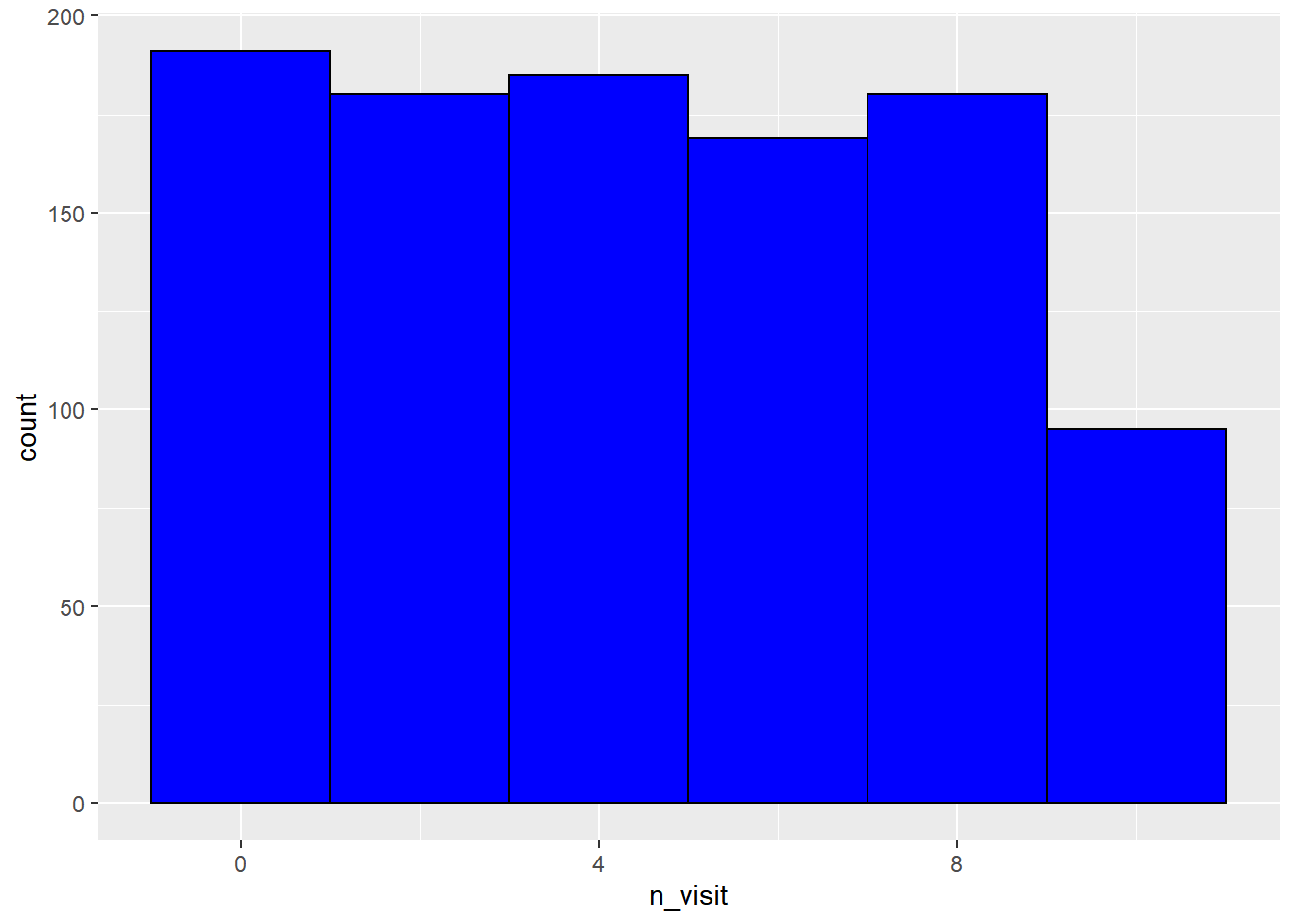## 10.7 Line Type

The line type of the histogram border can be modified using the `linetype` argument. It can take any integer value between `0` and `6`.

``````ggplot(ecom) +
geom_histogram(aes(n_visit), bins = 5, fill = 'white',
color = 'blue', linetype = 3)``````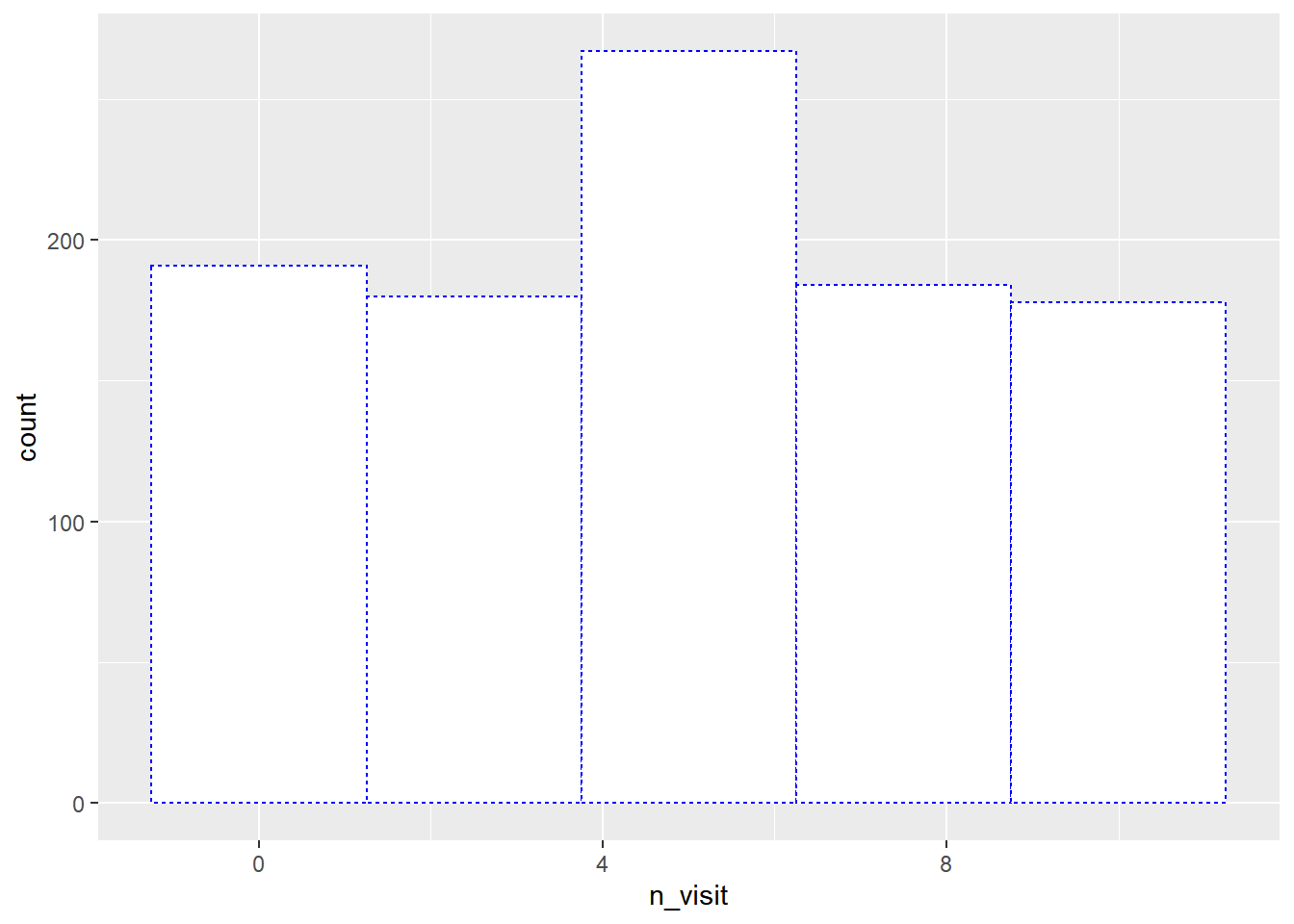## 10.8 Line Size

Use the `size` argument to modify the width of the border of the histogram bins. It can take any value greater than `0`.

``````ggplot(ecom) +
geom_histogram(aes(n_visit), bins = 5, fill = 'white',
color = 'blue', size = 1.25)``````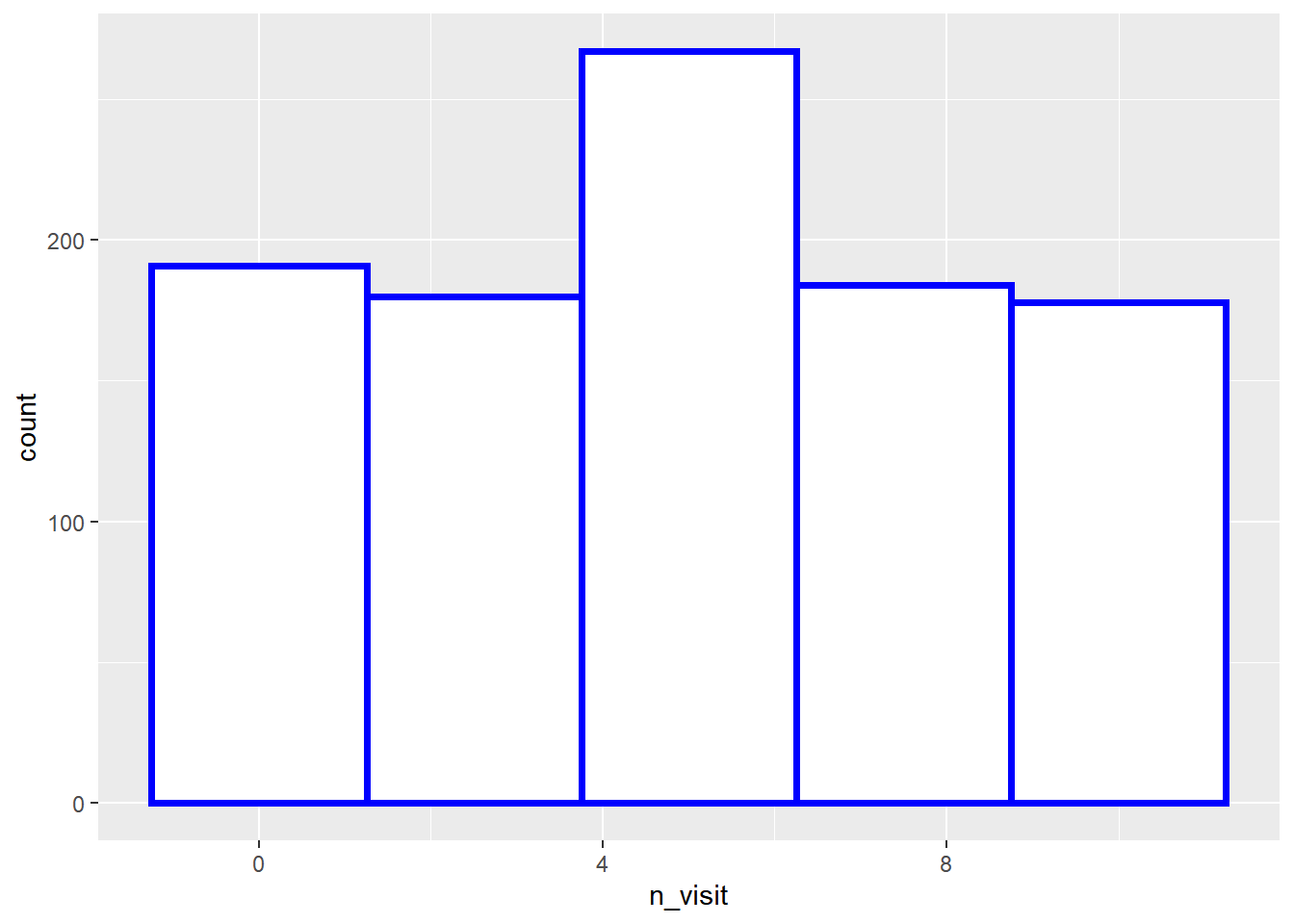## 10.9 Map Variables

You can map the aesthetics to variables as well. In the below example, we map `fill` to the device variable. You can try mapping color, linetype and size to variables as well.

``````ggplot(ecom) +
geom_histogram(aes(n_visit, fill = device), bins = 7)``````